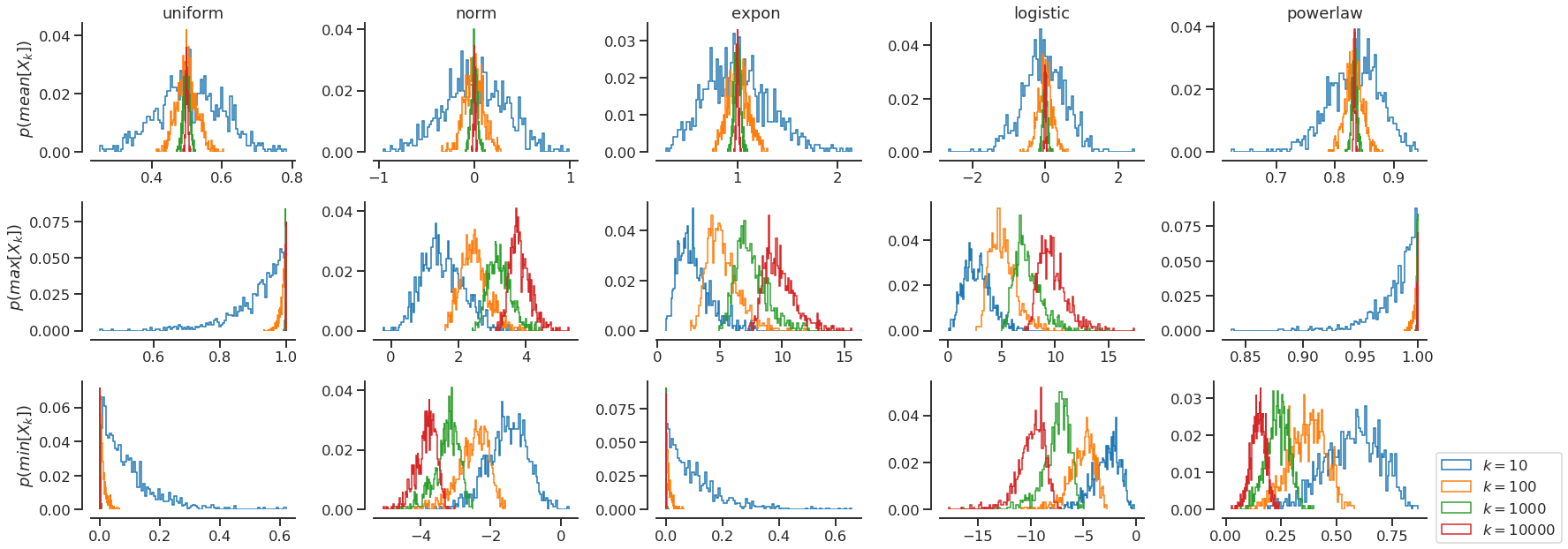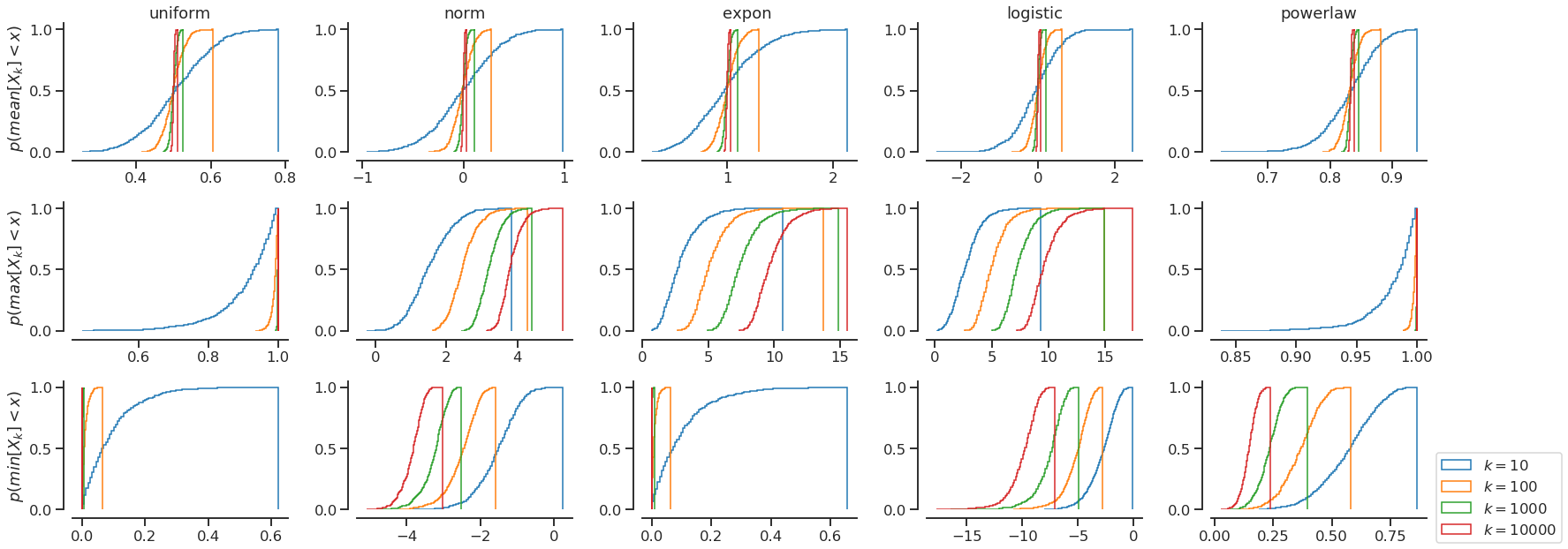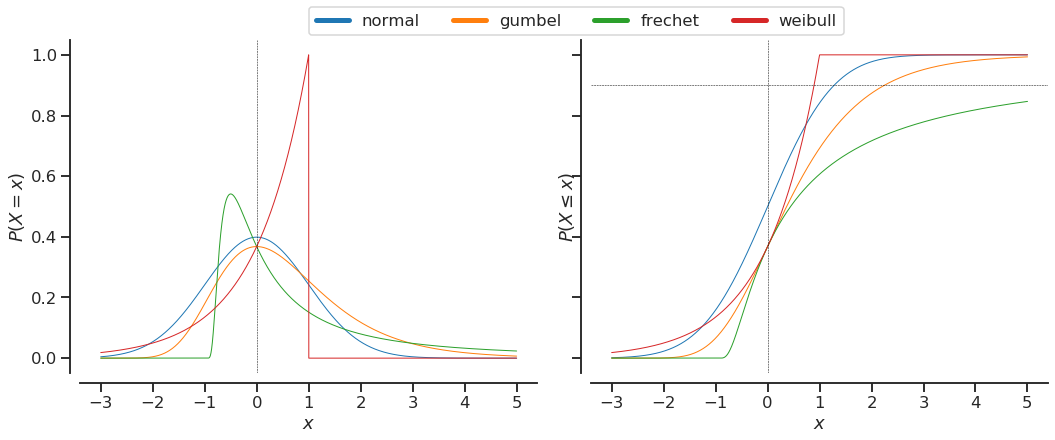# Part 1 - Understanding extreme value theory - Basics

Posted on 21/07/2019 in posts machine learning statistics

In :
%matplotlib inline

import numpy as np
import pandas as pd
from scipy import stats
import matplotlib.pyplot as plt
import seaborn as sns

In :
sns.set_context("talk")
sns.set_style("ticks")
np.random.seed(1337)


I will be writing about the Extreme value theory (EVT) which was introduced to me by my brother Sudhanshu, while he was working on his internship project. I really liked the connection it has with central limit theorem (CLT). The approach allowed me to better understand central limit theorem as a way to identify distribution of a function applied to independent and identically distributed (i.i.d.) samples. For CLT, the function is the mean or sum applied to the samples. For EVT the function is max or min.

I will do a multi part series trying to explain EVT using examples from real data. In the first part, the focus is on simply understanding the core ideas and introducing the limiting distributions for EVT.

Most of us know about the central limit theorem. It states that the sample means for i.i.d. samples from a distribution with finite variance, follows a normal distribution. More formally, we can write it as follows:

$$\begin{equation} X_1, X_2, ..., X_n \sim p(X)\\ S_n = \frac{\sum_i X_i}{n}\\ S_n \sim \mathcal{N}(\mu, \sigma^2)\\ \end{equation}$$

Similarly, there exists a the Extreme value theory. This theory deals with the distribution of the extreme values (like minimum or maximum) from the (i.i.d.) samples from some distribution.

$$\begin{equation} X_1, X_2, ..., X_n \sim p(X)\\ M_n = \max_i X_i\\ M_n \sim {\textrm {GEV}}(\mu ,\,\sigma ,\,\xi ) \\ \end{equation}$$

Surprisingly, like the central limit theorem, these values also converge on a class of distributions called the generalized extreme value distribution which characterizes three types of distributions. These distributions are:

Weibull law: $G(z)={\begin{cases}\exp \left\{-\left(-\left({\frac {z-b}{a}}\right)\right)^{\alpha }\right\}&z<b\\1&z\geq b\end{cases}}$ when the distribution of $M_{n}$ has a light tail with finite upper bound. Also known as Type 3.

Gumbel law: $G(z)=\exp \left\{-\exp \left(-\left({\frac {z-b}{a}}\right)\right)\right\}{\text{ for }}z\in \mathbb {R}$ . when the distribution of $M_{n}$ has an exponential tail. Also known as Type 1

Fréchet Law: $G(z)={\begin{cases}0&z\leq b\\\exp \left\{-\left({\frac {z-b}{a}}\right)^{-\alpha }\right\}&z>b.\end{cases}}$ when the distribution of $M_{n}$ has a heavy tail (including polynomial decay). Also known as Type 2.

In all cases, $\alpha >0$. [Source: https://en.wikipedia.org/wiki/Extreme_value_theory#Univariate_theory ]

The good thing about knowing the distribution of $M_n$ is that we can quantify what is the probability of observing a value as extreme as $M_n$. In case of maximum this can be computed by using the cumulative density function (CDF) as $P(M_n < x)$. If we set a threshhold on this probability (say $\delta = 99\%$) then we can make systems which are robust to the extreme value $\delta$ percentage of time. Also, we can identify an observation as a rare event if it has CDF more than $\delta$.

Let us see this in action. First we are going to create a draw_samples function which allows us to draw samples from various disitributions available in scipy.stats. We will draw samples of size $n$ and will draw $k$ such samples.

In :
def draw_samples(dist, n, k=1):
return dist.rvs(size=(n,k))

def plot_samples(dists, aggregations, n=1000, k_max=10000):
rows, cols = len(aggregations), len(dists)
fig, ax = plt.subplots(rows, cols, figsize=(5*cols, 3*rows))
fig_cum, ax_cum = plt.subplots(rows, cols, figsize=(5*cols, 3*rows))
for i, (dist_name, dist) in enumerate(dists):
samples = draw_samples(dist, n, k_max)
dist_mean = dist.mean()
dist_std = dist.std()
for j, (agg_name, agg) in enumerate(aggregations):
for k in [int(10**p) for p in np.arange(1, np.log10(k_max)+1)]:
normed_samples = samples[:, :k]
#normed_samples = (samples[:, :k] - dist_mean)/dist_std
X = agg(normed_samples, axis=1)
#print(dist_name, agg_name, k, X.shape)
ax[j, i].hist(
X, bins=100, weights=np.ones_like(X)/X.shape,
cumulative=False,
histtype="step",
label=f"$k={k}$"
)
ax_cum[j, i].hist(
X, bins=100, weights=np.ones_like(X)/X.shape,
cumulative=True,
histtype="step",
label=f"$k={k}$"
)
if j == 0:
ax[j, i].set_title(dist_name)
ax_cum[j, i].set_title(dist_name)
if i == 0:
ax[j, i].set_ylabel(f"$p({agg_name}[X_k])$")
ax_cum[j, i].set_ylabel(f"$p({agg_name}[X_k] < x)$")
if j == rows-1 and i == cols-1:
ax[j, i].legend(loc="best", bbox_to_anchor=(1.01, 0.5))
ax_cum[j, i].legend(loc="best", bbox_to_anchor=(1.01, 0.5))
sns.despine(fig=fig, offset=10)
sns.despine(fig=fig_cum, offset=10)
fig.tight_layout()
fig_cum.tight_layout()
return (fig, ax), (fig_cum, ax_cum)

In :
samples = draw_samples(stats.norm(), 100, 10000)
samples.shape

Out:
(100, 10000)

Let us compare the distribution of means, max, and min of samples from a few distributions distributions e.g. uniform, normal, exponential, logistic, and powerlaw. We are going to plot the PDF as well as the CDF of the distributions. The PDF tells us the neighborhood of high probability density, the CDF gives us a way to bound a certain percentage of the data.

In :
dists = [
("uniform", stats.uniform()),
("norm", stats.norm()),
("expon ", stats.expon()),
("logistic", stats.logistic()),
("powerlaw", stats.powerlaw(a=5)),
]

aggregations = [
("mean", np.mean),
("max", np.max),
("min", np.min),
]
plot_samples(dists, aggregations, n=1000, k_max=10000);As we can observe, as the number of samples $k$ increases, the estimation of the mean and the extreme values converges to the true value. However, unlike the central limit theorem, where the variance of estimated mean reduced for higher $k$, for extreme value distributions, the distribution shifts to either right (for max) or left (for min) as $k$ increases. The variance the extreme value distributions sometimes reduces or increases as $k$ increases. I am not aware of any theorem which proves the convergence of variance.

For bounded distributions like uniform, exponential, and powerlaw, the extreme value converges to the bound as $k$ increases. UPDATE: I found this very similar blog post https://eranraviv.com/distribution-sample-maximum/ which explains the convergence of sample maximum for a real use case of hiring top talent. I would highly recommend reading this.

## Comparing normal and GEV distributions¶

A good thing about these limiting GEV distributions is that they often fat tailed compared to the normal distribution, and also that they are skewed. This allows us to model extreme values more appropriately. We can observe this skew and fat tailed property by comparing normal distribution, to each of the GEV distributions.

In :
normal = stats.norm()
gumbel = stats.genextreme(c=0)
frechet = stats.genextreme(c=-1)
weibull = stats.genextreme(c=1)

In :
fig, ax = plt.subplots(1,2, sharex=True, sharey=True, figsize=(15, 6))

x = np.arange(-3, 5.001, 0.001)

ax.plot(x, normal.pdf(x), label="normal", lw=1)
ax.plot(x, gumbel.pdf(x), label="gumbel", lw=1)
ax.plot(x, frechet.pdf(x), label="frechet", lw=1)
ax.plot(x, weibull.pdf(x), label="weibull", lw=1)
ax.axvline(x=0, lw=0.5, linestyle="--", color="k")
ax.set_ylabel("$P(X=x)$")
ax.set_xlabel("$x$")

ax.plot(x, normal.cdf(x), label="normal", lw=1)
ax.plot(x, gumbel.cdf(x), label="gumbel", lw=1)
ax.plot(x, frechet.cdf(x), label="frechet", lw=1)
ax.plot(x, weibull.cdf(x), label="weibull", lw=1)
ax.axvline(x=0, lw=0.5, linestyle="--", color="k")
ax.axhline(y=0.9, lw=0.5, linestyle="--", color="k")
ax.set_ylabel("$P(X\leq x)$")
ax.set_xlabel("$x$")

lgd = fig.legend(
*ax.get_legend_handles_labels(),
bbox_to_anchor=(0.8, 1.05),
bbox_transform=fig.transFigure,
ncol=4
)

for lh in lgd.legendHandles:
lh.set_linewidth(5)

sns.despine(offset=10)
fig.tight_layout()As we can observe, each of the GEV distribution is suitable for data which is bounded on either side, or unbounded. Also, the tails of GEV distribitions are heavier compared to the normal distribution. This allows assigning higher probability to extreme values compared to normal distribution, thereby reducing the surprise on encoutering these values.

In future, parts we will see how we can fit GEV distributions to data and use it to identify outliers, or new classes.